## How to model laser beam propagation in OpticStudio Part 1 - Gaussian beam theory and ray-based approach.

Category: Product NewsIn a series of three blog posts, you will learn the key steps in modeling laser beam propagation in OpticStudio. Discover what tools are available, how to set up, analyze laser beam propagation, and optimize for the smallest beam size in a simple singlet lens system in OpticStudio sequential mode.

OpticStudio sequential mode provides three tools to model Gaussian beam propagation:

• Ray-based approach. It models beam propagation using geometrical ray trace.
• Paraxial Gaussian Beam. It models Gaussian beam and reports various beam data, including beam size and waist location as it propagates through a paraxial optical system.
• Physical Optics Propagation (POP). It models laser beams by propagating a coherent wavefront, which allows a very detailed study of arbitrary coherent optical beams.

This blog post starts with an overview of Gaussian beam theory and the terms and conventions used by OpticStudio. It sets up an example to show how to optimize a laser beam focusing system using a geometric ray-based approach.

Gaussian beam theory

Consider an ideal Gaussian beam with waist w0. As shown in the schematic below This Gaussian beam can be described using any two of the three parameters:

• wavelength λ
• beam waist w0
• divergence angle θThe beam size is a function of the distance from the waist. Please note that OpticStudio uses the half-width or radius to describe beam size.For large distances the beam size expands linearly. The divergence angle θ of the beam is given byHere, zR is the Rayleigh range of the beam given byThe phase radius of curvature of the beam is a function of the distance from the beam waist, z:This means that the radius is infinite at waist location z = 0, reaches its minimum of 2zR at z = zR, and asymptotically approaches infinity as z approaches infinity.

Ray-based approach to model Gaussian beam

Geometrical optics is the modeling of optical systems by tracing rays. Rays are imaginary lines which represent normals to the surfaces of constant phase, or the wavefront (see the Knowledgebase article "What is a ray?" for more details). For a paraxial Gaussian beam, within the Rayleigh range, z < zR, the beam size changes very slowly. In this case, beam can be modeled as collimated ray bundle. When far outside the Rayleigh range, z >> zR, beam size changes linearly with propagation distance, so beam can be modeled as a point source. This is shown in the drawings below.

As collimated ray bundle inside zR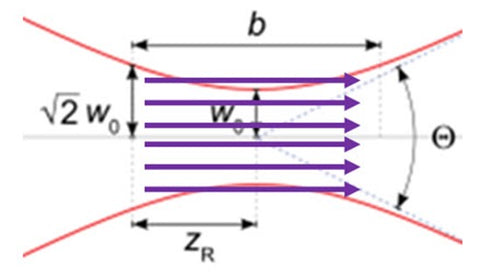As point source for z ≫ zRExample

In this example, we will set up a laser beam focusing system using a singlet lens and demonstrate how to optimize for the best focus using ray-based approach.

Suppose we have the following specifications of the laser from measured data:

• Nominal Wavelength = 355 nm
• Measured 5 mm from laser output:
• Beam diameter = 2 mm
• Measured divergence = 9 mradKnowing the wavelength and the far field divergence angle, using equations (1) through (3), the beam waist is calculated to be 0.0125 mm, with a Rayleigh range of 1.383 mm. We will focus the beam using a singlet lens. The goal is to optimize the system, so the beam size is the smallest at 100 mm away from the laser output. We will model this system using ray-based approach first.

As shown in the earlier section, when using rays to model Gaussian beam, we need to know if the propagation is within the Rayleigh range or outside Rayleigh range. This can help us decide if we should use a point source or a collimated ray bundle to model the beam. In this case, we know the beam waist location is inside of the Laser housing. Using equation (1), with the calculated beam waist 0.0125 mm and Rayleigh range 1.383 mm, we can manually calculate the beam propagation distance z from its waist, around 111.1 mm, when the beam hits the measurement location. Since this propagation distance is much larger than the beam Rayleigh range, z>>zR, we can then model the beam using a point source.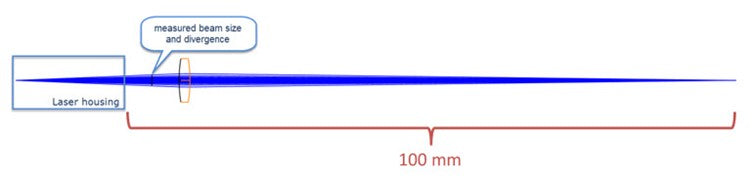The setup in OpticStudio is as follows:

In the System Explorer:

• The wavelength is set to 0.355 um.
• Aperture Type is enter Float By Stop Size with a Gaussian Apodization Type and Apodization factor of 1.0. In geometric ray tracing, the apodization factor can be used to produce a Gaussian type amplitude variation in the pupil to simulate illumination of the entrance pupil by a laser beam. Setting the apodization factor G=1.0 creates a Gaussian amplitude distribution where the beam intensity decreases to 1/e2 at the edge of the pupil. This means the beam width or radius is set to be the same as the semi-diameter of the entrance pupil.

In the Lens Data Editor:

• Object is at the laser beam waist location which lies a certain distance to the left of the laser output face.
• Surface 1 is the laser output face.
• Surface 2 is the STOP surface and 5 mm away from surface 1. This is the dummy surface where beam size and divergence angle were measured.• Using beam size equation (1), the waist location can be calculated first, and then the object plane can be set at the waist location.
• Alternatively, we could also make OpticStudio find the waist location for us, which is what we will do here. The Object Thickness value is 100 mm as a first guess andset as a variable.
• The Semi-Diameter value on the STOP surface 2 is 1 mm, to match the given 2 mm laser beam diameter.

The beam divergence is 9 mrad, measured at surface 2. This information can be entered in the Merit Function Editor using operand RANG, as shown below. RANG returns the ray angle in radians with respect to the local z axis of the specified surface. The Target is set to 9 mrad, and the Weight to 1.0.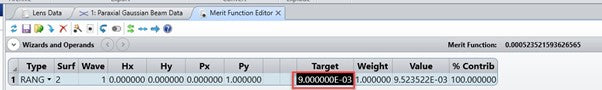When optimized the Object thickness becomes 106.108 mm. The marginal ray hits surface 2 at 9 mrad angle.

This indicates the ray-based approach placed the beam waist at 106.108 mm in front of (or to the left of) the laser output face surface 1.

Next is to optimize the singlet so it focuses the beam down to the smallest spot 100 mm away from the laser output face surface 1.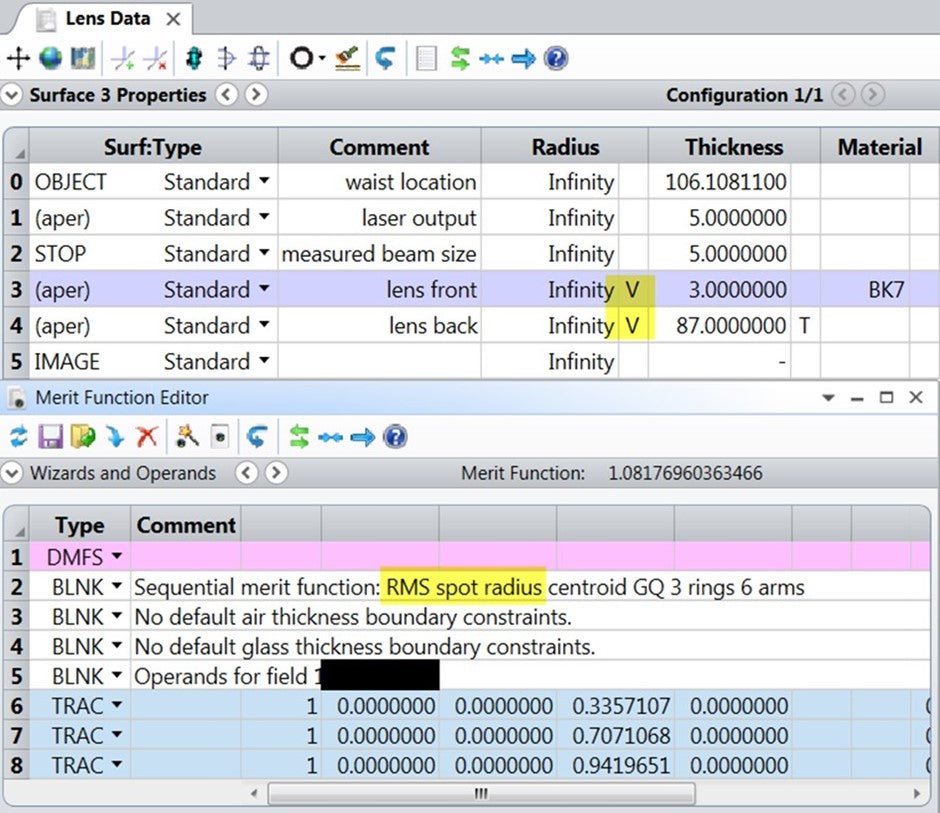After a quick local optimization run, the spot size decreases from initial 1.086 mm to 0.122 µm which is much smaller than the Airy disc radius 18.11 µm, reported by OpticStudio in the Standard Spot Diagram analysis window. This indicates the system is now diffraction limited. It is important to remember that in diffraction limited cases the RMS/Geometric spot radius is not a good measure of the real beam size (whether it is Gaussian or not) as it does not account for the effects of diffraction. OpticStudio provides other tools to study diffraction effect, for example the Fast Fourier Transform (FFT) PSF and Huygens PSF, as well as the Physical Optics Propagation (POP) tool. We will not discuss the FFT and Huygens PSF in this series, but we do describe how to use POP as a tool to focus this beam in an upcoming post.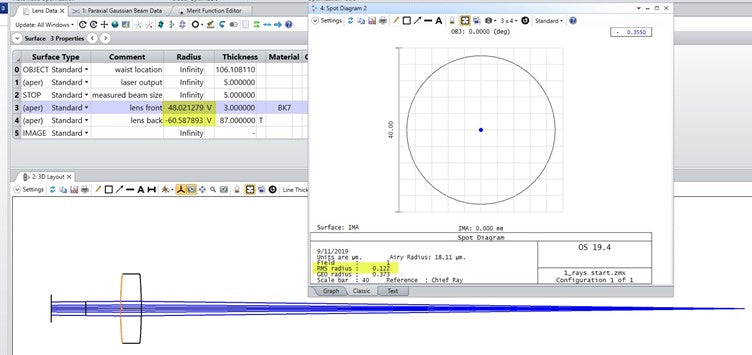To discover how well this result agrees with a Gaussian Beam calculation for a coherent laser beam, and to access example OpticStudio files, click here.

To learn how to model laser beam propagation in OpticStudio using Paraxial Gaussian Beam analysis check our blog homepage later this week.

Try Zemax optical design software capabilities for yourself, request a free trial.

Author:
Hui Chen
Senior Optical Engineer
Zemax an Ansys Company